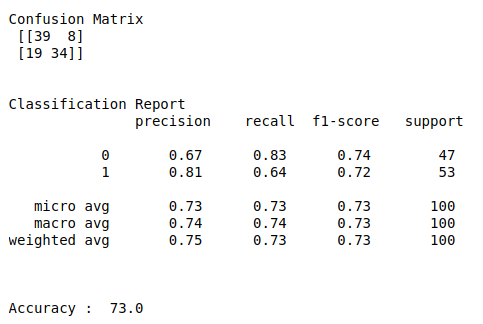• #5, First Floor, 4th Street Dr. Subbarayan Nagar Kodambakkam, Chennai-600 024 Landmark : Samiyar Madam
• pro@slogix.in
• +91- 81240 01111

#### Social List

##### Sentiment analysis on amazon products reviews using Decision tree algorithm in python?
###### Description

To train a machine learning model for classify products review using Decision tree in python.

#### Input

Amazon product review data set. (Kaggle)

#### Output

Confusion matrix, classification report and accuracy_score.

###### Process

Import library.

Declare independent and dependent variables.

Do pre process for text data.

Split the data into train and test.

Build the model.

Fit the train data into the model.

Evaluate the model using test data.

###### Sapmle Code

#import necessary libraries
import pandas as pd
import numpy as np
import re
import warnings
import seaborn as sns
warnings.filterwarnings(“ignore”)
import matplotlib.pyplot as plt
from sklearn.model_selection import train_test_split
from sklearn.tree import DecisionTreeClassifier
from sklearn.feature_extraction.text import TfidfVectorizer
from sklearn.metrics import classification_report, confusion_matrix, accuracy_score

#check missing values
print(“Checking missing values\n\n”)
print(data.isnull().sum())

#make it as a data frame
df = pd.DataFrame(data)

#counts in each class
print(“\n”)
print(“Counts in each class\n”)
print(df[‘type’].value_counts())

#Features
y = df[‘type’]

#change text lower cases and removal of white spaces
lower_text = []
for i in range(0,len(X)):
s = str(X[i])
s1 = s.strip()
lower_text.append(s1.lower())
#print(“After converting text to lower case\n\n”,lower_text)

#Remove punctuation
punc_text = []
for i in range(0,len(lower_text)):
s2 = (lower_text[i])
s3 = re.sub(r'[^\w\s2]’,”,s2)
punc_text.append(s3)
#print(“After removed punctuation\n\n”,punc_text)

#Word vectorization
#Initialize the TF-IDF vectorizer
tfidf = TfidfVectorizer(sublinear_tf=True, min_df=5,max_df = 0.7,norm=’l2′, encoding=’latin-1′, ngram_range=(1, 2),
stop_words=’english’)

#transform independent variable using TF-IDF vectorizer
print(“\n”)
X_tfidf = tfidf.fit_transform(punc_text)
print(“After vectorized text data\n\n”,X_tfidf)

#Split the data into train and testing
X_train, X_test, Y_train, Y_test = train_test_split(X_tfidf, y, test_size=0.1, random_state=0)

#Print training data
print(“\n”)
print(“Training data\n\n”,X_train,”\n”,Y_train)
print(“\n\n”)

#Print testing data
print(“Testing data\n\n”,X_test)
print(“\n\n”)

#Build the Decision tree model
clf = DecisionTreeClassifier()

#Fit train and test into the model
clf.fit(X_train, Y_train)

#Predict the result
y_pred = clf.predict(X_test)

#classification report & confusion matrix
print(“Confusion Matrix\n”,confusion_matrix(Y_test,y_pred))
print(“\n”)
print(“Classification Report\n”,classification_report(Y_test,y_pred))
print(“\n”)
print(“Accuracy : “,accuracy_score(Y_test,y_pred)*100)

###### Screenshots